# Taylor Dispersion Analysis (TDA) Introduction and Overview

Taylor Dispersion Analysis (TDA), or Taylor-Aris dispersion, is a technique for rapidly determining the diffusion coefficients of molecules. From the diffusion coefficient values, hydrodynamic radii of the molecules can be determined. Taylor described the TDA method for the first time in his classic paper, which covered the measurement of the dispersion of an injected solute pulse into a flowing fluid in a capillary. The method was further developed by Aris when he took into account the longitudinal diffusion of the molecules.

According to Taylor, convection as a result of the fluid’s cross-sectional velocity and the radial diffusion of the molecules make the solute to disperse. The flow of a pressure-driven fluid in a cylindrical tube under Poiseuille laminar conditions experiences a drop in the velocity of the fluid radially from a maximum, umax, at the center of the cylinder to a minimum, umin, at the cylinder walls. Figure 1 shows the effect of this velocity distribution on the profile of an injected solute pulse.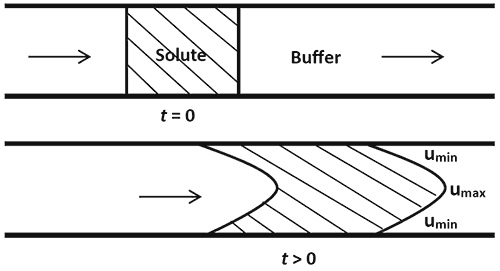Figure 1. Axial spreading of the solute due to convection.

The solute pulse spreads axially along the direction of flow, which is known as convection. The pulse’s front and back ends start out as sharp interfaces, becoming parabolic in shape because of the laminar flow of the fluid. Without any other dispersion mechanism, the axial spreading would continue over time, increasingly dispersing over the length of the tube and resulting in an asymmetric concentration distribution.

Diffusion is often considered to be a spreading mechanism due to the transfer of solute molecules from higher concentration regions to lower concentration regions. Conversely, in Taylor Dispersion, radial diffusion limits the axial spreading because of convection (Figure 2).Figure 2. The role of radial diffusion.

At the pulse’s front end, the concentration of the solute is greater at the cylinder center than at the walls of the cylinder, leading to radial diffusion of the solute molecules towards the cylinder walls. However, diffusion reduces the average axial velocities of solute molecules, as the movement of the solute molecules is much faster at the center than at the cylinder walls.

Conversely, the concentration of the solute is more at the walls than at the center in the pulse’s rear end, leading to radial diffusion of the solute molecules towards the center. This, in turn, increases the average axial velocities of the diffusing solute molecules. In general, the role of the radial diffusion is to speed up the trailing interface, but slow down the leading interface. This enables more compact solute distribution in the axial direction, when compared to convection alone.

The axial spreading due to both radial diffusion and convection allows the injected solute pulse to develop a symmetrical concentration distribution, which is called Taylor Dispersion. The diffusion of solute molecules also occurs in a direction parallel to the flow. This contribution is much less than that of the overall axial dispersion. This is called Taylor-Aris dispersion.

## Taylor Dispersion Analysis

The amount of solute dispersion in a solvent stream is inversely related to the diffusion coefficient of the solute. The amount of dispersion can be represented as a function of the time elapsed and the position of the solute in the flow: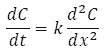(1)

Where C = solute concentration averaged across the capillary diameter in the y-direction and at a right angle to the flow axis x (Figure 3) and k = dispersion coefficient, which is correlated to the diffusion coefficient (D) as follows: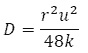(2)

Where r = capillary radius and u = mean speed of the fluid flow.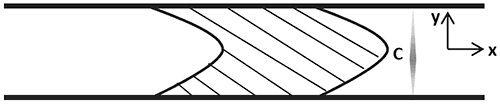Figure 3. The concentration is averaged over the y-direction, perpendicular to the flow axis x.

There is an inverse relationship between the hydrodynamic radius (Rh) and diffusion coefficient as expressed by Stokes' law: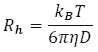(3)

Where kB = Boltzmann's constant, T = temperature and h = solvent viscosity.

Depending on the initial conditions, Equation 1 has a range of possible solutions describing the evolution of the concentration profile as a function of time and space (Figure 4).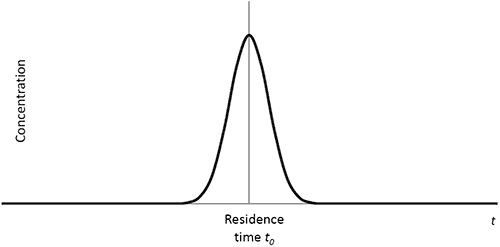Figure 4. The concentration profile resulting from the injection of a small nanoliter-scale sample pulse.

The concentration profile (or Taylorgram) is obtained during the initial concentration of the sample within a small region at point x = 0. The concentration, C, at a point x and at time t along the capillary is expressed as: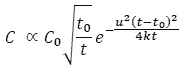(4)

Where C0 = initial sample concentration and t0 = x/u (residence time). The residence time is roughly equal to the time of peak concentration (Figure 4). Equation 4 is known as the time-dependent Gaussian distribution and it can be approximated by a Gaussian distribution at large values of t using: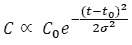(5)

Where the dispersion coefficient k is correlated to the width of the Gaussian ‘s’ as follows: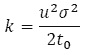(6)

Equations 4 and 5 are based on the assumption that C0 is within an infinitesimally small region at the point x = 0, which is practically not possible. Therefore, a correction is introduced to Equation 6 by considering the finite width of the injected sample. It is possible to avoid the need for the correction by measuring the dispersion at two points along the capillary. This is because we can deduce the dispersion coefficient through a comparison of the evolution of the dispersion between the two observation points. The dispersion coefficient can be related to the widths of the Gaussians in the following way: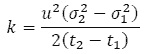(7)

This is generally known as the two-window solution.

It is necessary to satisfy two other conditions for the solutions in Equations 2, 4, 5 and 7 to be valid. The observation time t must be much higher than the time needed for the radial diffusion of the solute molecules across the capillary, which can be expressed in terms of the dimensionless residence time t: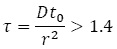(8)

Moreover, the axial diffusion of the solute must be insignificant in comparison with its dispersion: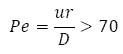(9)

Where Pe = the Peclet number.

Since the Taylor-Aris dispersion accounts for the effect of axial diffusion, it does not require the second condition. The Taylor-Aris solution amounts to substituting Equation 2 with Equation 10: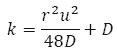(10)

## TDA with UV-Selective Detection Using Malvern Panalytical Viscosizer

The Malvern Panalytical Viscosizer system can rapidly determine hydrodynamic radius of a molecule, enabling mass-weighted sizing of small molecule and protein-based systems in biological formulations using TDA with UV-selective detection.

Additionally, relative viscosity measurements can be performed in microcapillary flow on the same sample with the Malvern Panalytical Viscosizer system to complement these TDA-based measurements. Very small sample volumes are enough to run all test protocols under automated methodologies.

Molecular sizing by TDA will be optimal for hydrodynamic radius in the range of 0.2-50 nm. An ultra-violet (UV) light imaging array is used to measure the absorbance of an injected sample as a function of time, as it is driven by pressure through a narrow bore fused-silica capillary with two detection windows.

For size measurements, the capillary diameter is around 75 mm and the typical run pressure is 140 mb. These values, along with the positions of the two detection windows, have been selected to satisfy the two aforementioned conditions for a broad range of typical hydrodynamic radii. The run buffer is used to set the measurement baseline.

TDA can baseline against the run buffer and evaluate the size and behavior of target molecules in the injected sample pulse selectively, paving the way for new experimental capabilities to perform label-free analysis in complicated, biologically-relevant matrices such as monitoring drug molecules in serum. Moreover, the ability of the system to determine the viscosity of such complex sample matrices provides meaningful complementary data for diffusion coefficient.

Figure 5 shows a schematic of the measurement set-up, and Figure 6 depicts the absorbance profiles obtained.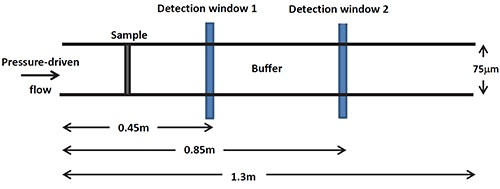Figure 5. A schematic of the Malvern Panalytical Viscosizer measurement set-up.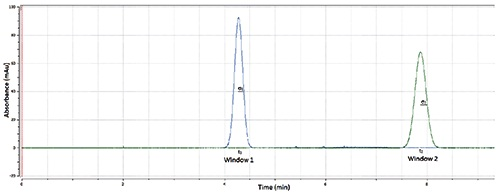Figure 6. A Taylorgram showing the absorbance profiles at the two detection windows.

The UV absorbance A of a sample is related to its concentration C by the Beer-Lambert law as follows: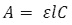(11)

Where e = Extinction coefficient, which depends on the molecule and is the path length of the light through the sample. Therefore, the absorbance profile of a sample varies in proportion to the distribution of its concentration. The dispersion of the sample within the capillary can be measured using its absorbance profile.

The absorbance profile is fitted with a model based on Equation 4 to obtain the width s and residence time t0 of a Taylorgram using the Malvern Panalytical Viscosizer. k is determined using the two-window solution (Equation 7), from which D and Rh of the sample (Equations 2 and 3) are estimated.

Besides homogenous samples, multi-component mixtures such as monomer and dimer or other oligomeric species, or aggregated samples can be analyzed using the Taylor Dispersion method. In this case, the estimated hydrodynamic radius from a single fit to the Taylorgram is a weighted average value of the individual hydrodynamic radii. Therefore, changes in the calculated hydrodynamic radii are an indication of a corresponding change in the amount of oligomers or aggregates in a sample.

To obtain optimized results, individual fitting of the contributions to the Taylorgram of the each constituent of the sample is carried out to estimate the radii and relative proportions of the components from the widths and areas of the component Taylorgrams. The amount of oligomerisation or aggregation in the injected sample is then quantified using the results.This information has been sourced, reviewed and adapted from materials provided by Malvern Panalytical.

## Citations

• APA

Malvern Panalytical. (2019, September 03). Taylor Dispersion Analysis (TDA) Introduction and Overview. AZoM. Retrieved on September 25, 2020 from https://www.azom.com/article.aspx?ArticleID=12173.

• MLA

Malvern Panalytical. "Taylor Dispersion Analysis (TDA) Introduction and Overview". AZoM. 25 September 2020. <https://www.azom.com/article.aspx?ArticleID=12173>.

• Chicago

Malvern Panalytical. "Taylor Dispersion Analysis (TDA) Introduction and Overview". AZoM. https://www.azom.com/article.aspx?ArticleID=12173. (accessed September 25, 2020).

• Harvard

Malvern Panalytical. 2019. Taylor Dispersion Analysis (TDA) Introduction and Overview. AZoM, viewed 25 September 2020, https://www.azom.com/article.aspx?ArticleID=12173.